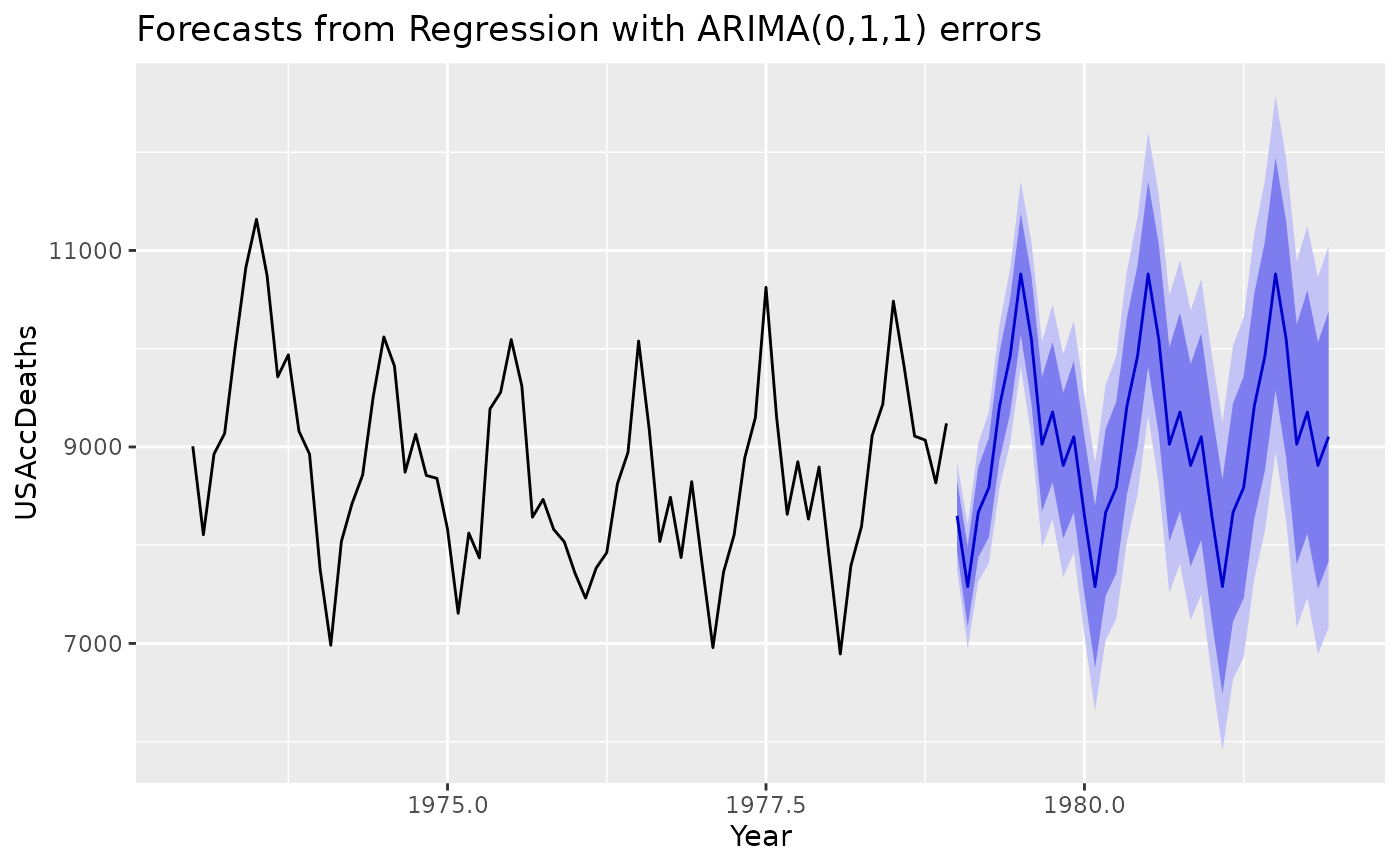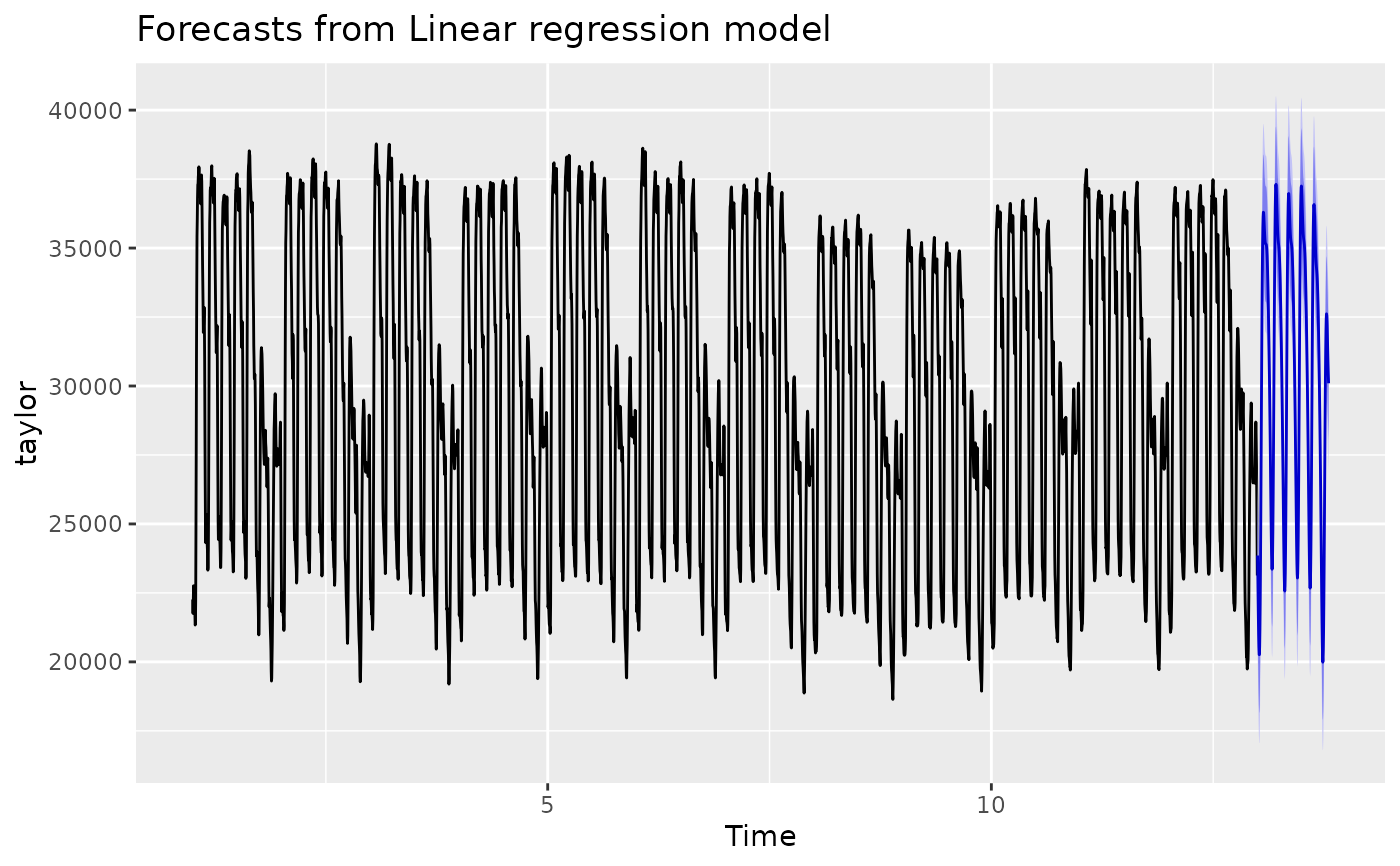fourier returns a matrix containing terms from a Fourier series, up to order K, suitable for use in Arima, auto.arima, or tslm.

fourier(x, K, h = NULL)

fourierf(x, K, h)

## Arguments

x

Seasonal time series: a ts or a msts object

K

Maximum order(s) of Fourier terms

h

Number of periods ahead to forecast (optional)

## Value

Numerical matrix.

## Details

fourierf is deprecated, instead use the h argument in fourier.

The period of the Fourier terms is determined from the time series characteristics of x. When h is missing, the length of x also determines the number of rows for the matrix returned by fourier. Otherwise, the value of h determines the number of rows for the matrix returned by fourier, typically used for forecasting. The values within x are not used.

Typical use would omit h when generating Fourier terms for training a model and include h when generating Fourier terms for forecasting.

When x is a ts object, the value of K should be an integer and specifies the number of sine and cosine terms to return. Thus, the matrix returned has 2*K columns.

When x is a msts object, then K should be a vector of integers specifying the number of sine and cosine terms for each of the seasonal periods. Then the matrix returned will have 2*sum(K) columns.

seasonaldummy

Rob J Hyndman

## Examples


library(ggplot2)

# Using Fourier series for a "ts" object
# K is chosen to minimize the AICc
deaths.model  <- auto.arima(USAccDeaths, xreg=fourier(USAccDeaths,K=5), seasonal=FALSE)
deaths.fcast <- forecast(deaths.model, xreg=fourier(USAccDeaths, K=5, h=36))
autoplot(deaths.fcast) + xlab("Year")# Using Fourier series for a "msts" object
taylor.lm <- tslm(taylor ~ fourier(taylor, K = c(3, 3)))
taylor.fcast <- forecast(taylor.lm,
data.frame(fourier(taylor, K = c(3, 3), h = 270)))
autoplot(taylor.fcast)